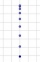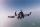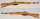# Free fall

How long does the stone fall freely into a depth of 80m? What speed will it hit the bottom of the abyss?

t =  4.0386 s
v =  39.6182 m/s

### Step-by-step explanation:Did you find an error or inaccuracy? Feel free to write us. Thank you!Tips to related online calculators
Do you want to convert velocity (speed) units?
Do you want to convert time units like minutes to seconds?

## Related math problems and questions:

• AbyssThe stone fell into the abyss: 2 seconds after we heard hitting bottom. How deep is the abyss (neglecting air resistance)? (gravitational acceleration g = 9.81 m/s2 and the speed of sound in air v = 343 m/s)
• Free fallFor how long and at what speed does the body fall to the ground during a free fall from a height of 35 m?
• Up and down motionWe throw the body from a height h = 5 m above the Earth vertically upwards v0 = 10 m/s. How long before we have to let the second body fall freely from the same height to hit the Earth at the same time?
• Free fallThe free fall body has gone 10m in the last 0.5s. Find the body speed at the moment of impact.
• Free fallLloyd fall from height 7 m. Calculate the speed he hit the ground when falling with acceleration g = 9.81 m/s2
• AccelerationThe car accelerates at a rate of 0.5 m/s2. How long travels 400 meters, and what will be its speed?
• Free fallThe elevator car weighing 720 kg is torn off at a height of 125 m and begins to fall in a free fall. How much kinetic energy does the elevator car have when it hits the ground floor of the building, when the free fall of the car lasted 5 s?
• GravitationFrom the top of the 80m high tower, the body is thrown horizontally with an initial speed of 15 m/s. At what time and at what distance from the foot of the tower does the body hit the horizontal surface of the Earth? (use g = 10 ms-2)
• Acceleration 2if a car traveling at a velocity of 80 m/s/south accelerated to a velocity of 100 m/s east in 5 seconds, what is the cars acceleration? using Pythagorean theorem
• BrakesThe braking efficiency of a passenger car is required to stop at 12.5 m at an initial speed of 40 km/h. What is the acceleration braking by brakes?
• Free fall 5Jeremy accidently dropped his toy stuffed animal from the balcony of his apartment on the fourth floor. The toy hit the ground at a velocity of 16.0 meters/second. At impact, it took 2.0 seconds for the toy’s velocity to reach 0 meters/second. If the toy
• The projectileThe projectile was fired horizontally from a height of h = 25 meters above the ground at a speed of v0 = 250 m/s. Find the range and flight time of the projectile.
• The carThe car weight 1280 kg, increased its speed from 7.3 m/s to 63 km/h on a track of 37.2 m. What force did the car engine have to exert?
• BomberThe aircraft flies at an altitude of 4100 m above the ground at speed 777 km/h. At what horizontal distance from the point B should be release any body from the aircraft body to fall into point B? (g = 9.81 m/s2)
• The projectileThe projectile leaves the 10 m long barrel at an instantaneous speed of 500 m/s. What is the acceleration of the movement of the projectile in the barrel and in what time will the projectile pass the barrel if, we assume that the movement was evenly accel
• A carA car weighing 1.05 tonnes driving at the maximum allowed speed in the village (50 km/h) hit a solid concrete bulkhead. Calculate height would have to fall on the concrete surface to make the impact intensity the same as in the first case!
• CollisionThe two bodies, whose initial distance is 240 m, move evenly against each other consistently. The first body has an initial velocity of 4 m/s and an acceleration of 3 m/s2, the second body has an initial speed of 6 m/s and an acceleration of 2 m/s2. Fin